# Edit

August 19, 2019: This post is severely outdated. Since the 1.0.0 release, I’d recommend to use the NumPy/SciPy class over the TensorFlow class for the vast majority of use cases. The 1.0.0 release introduced a number of improvements to the NumPy/SciPy class that make the following benchmarks obsolete.

The upcoming 0.5.0 version of pwlf has introduced a number of changes. The most notable change is the addition of a PiecewiseLinFitTF object, which will be available if you have TensorFlow installed. This post will benchmark the performance of this new object vs the older Numpy/SciPy class.

# Why TensorFlow

I ported the fitting class of pwlf to TensorFlow because I wanted to see if some of the optimizers in TensorFlow would be an improvement for finding breakpoints, especially in the presence of a large amount of data. While I have yet to explore different optimization methods, I did notice that the TensorFlow port was faster with larger amounts of data.

TensorFlow’s lstsq defaults to using a Cholesky decomposition to perform a least squares fit. The Cholesky decomposition will be faster than the Numpy/Scipy default, which uses a divide-and-conquer singular value decomposition (SVD). The tradeoff here is speed vs numerical stability. Note that setting the optional parameter fast=False will result in a complete orthogonal factorization, which would be the Numpy/SciPy equivalent of using lapack_driver=’gelsy’.

Now the performance of pwlf for fitting with known breakpoint locations is largely dependant on the least squares routine. However, it also depends on the time to assemble the linear regression matrix. The assembly in PiecewiseLinFitTF is slightly different than PiecewiseLinFit, due to the differences between Numpy and TensorFlow. Thus comparing the run time in pwlf between the two classes won’t just be a least squares benchmark.

# Benchmark

I’ve created a benchmark problem to compare the performance of the new TensorFlow pwlf class against the original class. The benchmark performs fits with known breakpoint locations for 6 and 20 line segments. The code to perform the benchmarks is available here. The number of data points was varied from 1e3 to 1e7, and 10 replicate fits were performed for each configuration. The benchmarks were run on the following computers:

CPU OS TF & SciPy built from source
AMD FX-8350 Linux Yes
Intel i5-6300u Linux Yes
AMD Ryzen 7 2700x Windows No

The results below compare the run time of the fits against the number of data points. The standard method refers to the old PiecewiseLinFit class, while TF CPU refers to the new PiecewiseLinFitTF class. One of the additions to the TensorFlow port is the ability to use single precision with the optional parameter dtype=’float32’. Note that the Cholesky decomposition routinely failed on the Windows machine with tensorflow.version == 1.13.1. I didn’t have this issue on the linux machines, which used the latest 1.13.1 TensorFlow source. The vertical error bars denote the 10th and 90th percentile, considering a Normal distribution with 90% confidence (from the 10 replicate runs).

# the benchmark basically performs the following
import numpy as np
import pwlf
from time import time
breaks = np.linspace(0.0, 10.0, num=21)
# generate sin wave data
x = np.linspace(0, 10, num=n_data)
y = np.sin(x * np.pi / 2)
# add noise to the data
y = np.random.normal(0, 0.05, size=n_data) + y
# normal PWLF fit
t0 = time()
my_pwlf = pwlf.PiecewiseLinFit(x, y)
ssr = my_pwlf.fit_with_breaks(breaks)
t1 = time()
# PWLF TF fit
t2 = time()
my_pwlf = pwlf.PiecewiseLinFitTF(x, y)
ssr = my_pwlf.fit_with_breaks(breaks)
t3 = time()


# 6 line segment results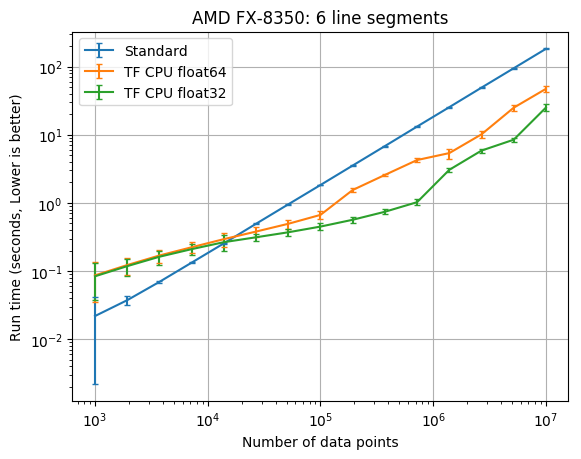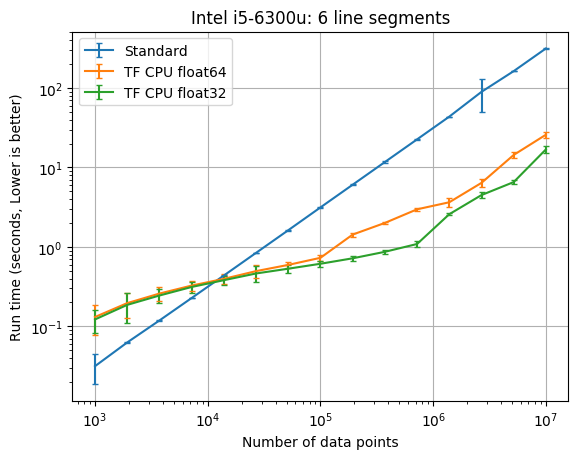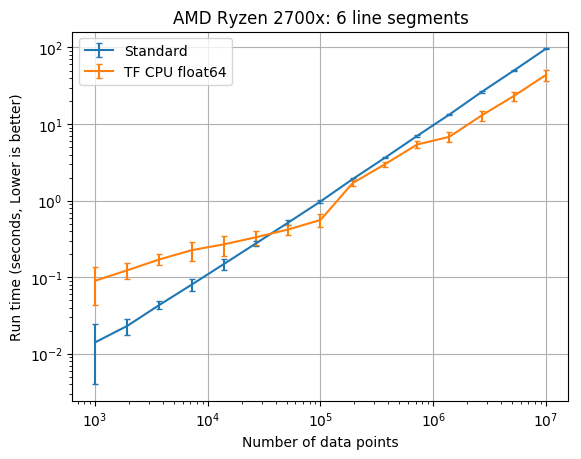CPU TF dtype TF times faster than SciPy with 1e7 data
AMD FX-8350 float32 7.3
AMD FX-8350 float64 3.9
Intel i5-6300u float32 18.6
Intel i5-6300u float64 12.2
AMD Ryzen 7 2700x float64 2.2

# 20 line segment results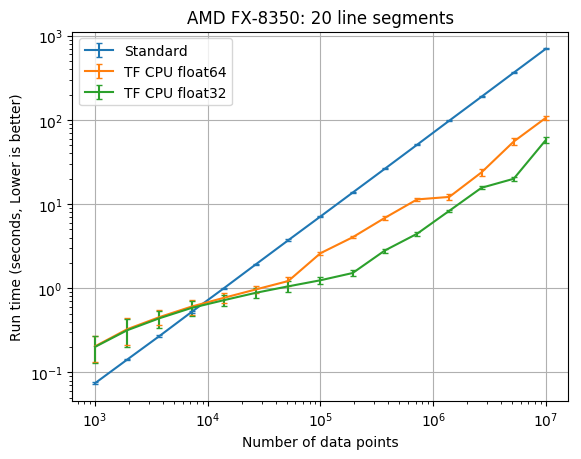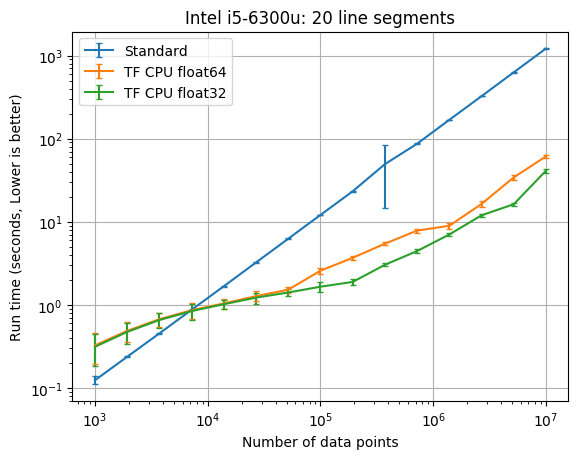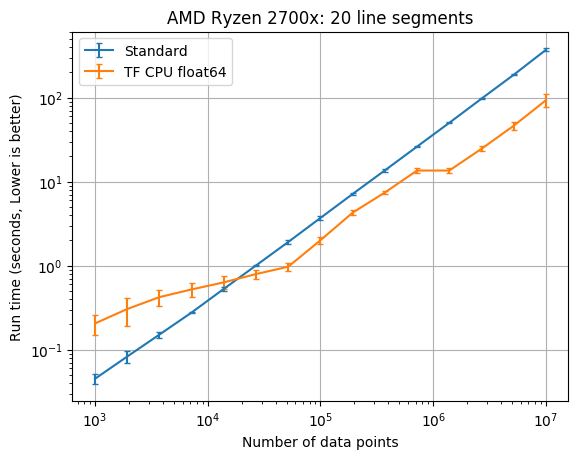CPU TF dtype TF times faster than SciPy with 1e7 data
AMD FX-8350 float32 12.1
AMD FX-8350 float64 6.6
Intel i5-6300u float32 29.5
Intel i5-6300u float64 19.8
AMD Ryzen 7 2700x float64 4.0

# Conclusion

You should start considering the new TensorFlow class when you have at least 20,000 data points. The PiecewiseLinFitTF class was somewhere between 2 times and 12 times faster than the original pwlf class with 10,000,000 data points. The difference here will greatly depend upon your CPU. If you don’t require a double precision floating point model, you should also consider using the float32 data type which was between 4 and 30 times faster than the original pwlf implementation. While I only tested the fit_with_breaks function, the speed up should be the same with the fit and fitfast functions. While the TensorFlow class was faster with larger amounts of data, it was usually much slower than Numpy/SciPy for small data applications.

# Miscellaneous Notes

• Numpy, SciPy, TF were built from source on Linux
• Intel mkl was used as the Numpy and SciPy backends on all machines (This isn’t great for AMD, but until BLIS comes out this is the best available…)
• TensorFlow with the low voltage Intel i5 was much faster than the AMD machines